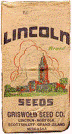## Extension## Historical Materials from University of Nebraska-Lincoln Extension

#### Title

EC872 Crop Budgets: Nebraska - 2014

2014

#### Citation

EC872 Crop Budgets Nebraska – 2014

© 2014, The Board of Regents of the University of Nebraska on behalf of the University of Nebraska–Lincoln Extension.

#### Abstract

This publication contains 66 crop production budgets for 13 crops, as well as tables of power, machinery, labor, and input costs used to develop these budgets. Each budget consists of five sections: • Heading • List of representative field operations • List of materials and services used • Operations and interest tabulations • Overhead costs including real estate taxes and opportunitycharges The budgets are presented in a worksheet format with a “Your Estimate” column for recording cost modifications.

Budget Divisions The heading consists of the crop name, system description, and method of water application. The list of representative field operations is organized in a table with columns for the operation name, quantity or number of times used with units, labor, fuel and lube, power source, and implement costs for both repairs and ownership. “Times” or “Quantity” is typically in acres with a decimal denoting where an operation is done on a fraction of acres or where it represents the probability of an operation being done. Those operations that are done multiple times, for example swathing several cuttings of hay, show the number of times. If a unit is other than “acres,” it is specified in the “Unit” column. Other units used are bushels (bu), hundredweight (cwt), tons, and acre-inches (ai).

Labor costs for each operation were calculated from machinery accomplishment rates and adjusted for additional time required for getting machinery ready, adjusting machinery, and handling fertilizer and other supplies. The estimated costs for completing these operations are multiplied by the number in the “Times” or “Quantity” column, the product of which is multiplied by the hourly wage (\$20 per hour) and labor factor.

Fuel costs also use machinery accomplishment rates as well as estimated fuel consumption rates to determine fuel use. This is multiplied by a lube factor and the price of energy which is \$3.50 per gallon for diesel and \$0.10 per kwh for electricity. Repairs and depreciation costs were estimated using functions and factors from the AgriculturalEngineer’s Yearbook which is published by the American Society of Agricultural and Biological Engineers. It requires making assumptions about the size and age of the equipment. It was assumed that machinery chosen was fully utilized.

Data used to calculate power unit costs are in Table 1 and machinery operation costs are in Table 2. Irrigation costs were calculated using engineering performance standards and typical water application rates which will depend on the rainfall area. Power costs for irrigation refer to the pump and power unit; implement costs are for the delivery system (pipe or pivot). Depreciation and interest for the well are budgeted with land costs. Materials and services are calculated by multiplying the application rate by the application price (Table 3) and then by the percent acres applied. A value less than 100% is used when a material or service is applied on only part of the acres or part of the time. For example, fields planted with Bt corn seed must have 20 percent of the acres planted to a refuge crop. There would be 20 percent in the column called “Percent Acres Applied” for the non- Bt seed and 80 percent for the Bt seed. Another example is when a practice is not always used. If an insecticide is used one year out of four, a “25 percent” would be entered in the column “Percent Acres Applied.” The cost for each material/service is computed by multiplying the percentage of acres by the quantity per acre and then by the price per unit. Note: All prices for materials and services in the budgets were obtained in October 2012. The value in the “Operation Index” column in the “Materials and Services” section indicates the corresponding operation in the “Field Operations” section. Data for calculating materials cost is in Table 3. The operations and interest is the sum of totals of the first two sections with interest calculated on the cash costs. Cash costs in interest calculations include labor, fuel, and repairs from the list of field operations and all costs from the materials and services. Overhead costs include accounting, liability insurance, vehicle cost, and office expense. Real estate values used are from the UNL publication Nebraska Farm Real Estate Market Developments 2012-2013 published in June 2012. Taxes on real estate are not included in interest calculations because in Nebraska they are due at the end of the year in which they accrue and are not delinquent until May and September of the following year. A cost per unit of production and cash cost per unit of production is calculated. The cost per unit of production is the sum of all costs divided by the projected yield. The cash cost per unit of production is the sum of labor, fuel, repairs, materials and services, and interest, divided by the projected yield. It should be noted that many enterprise budgets are cost and return estimates. These budgets are cost estimates only so have no estimates as to profitability.

COinS